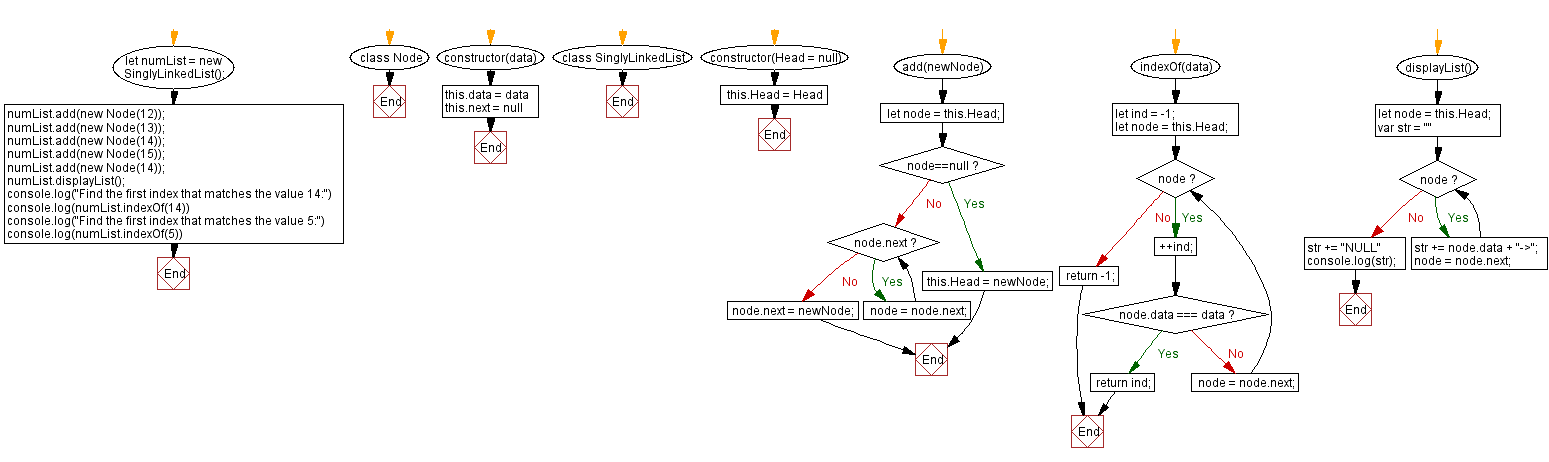# JavaScript Exercises: Find the first index that matches a given element

## JavaScript Singly Linked List: Exercise-8 with Solution

Write a JavaScript program to find the first index that matches a given element. Return -1 for no matching.

Sample Solution:

JavaScript Code:

``````class Node {
constructor(data) {
this.data = data
this.next = null
}
}
}
if(node==null){
return;
}
while (node.next) {
node = node.next;
}
node.next = newNode;
}
indexOf(data){
let ind = -1;
while(node){
++ind;
if(node.data === data) return ind;
node = node.next;
}
return -1;
}
displayList(){
var str = ""
while (node) {
str += node.data + "->";
node = node.next;
}
str += "NULL"
console.log(str);
}
}
numList.displayList();
console.log("Find the first index that matches the value 14:")
console.log(numList.indexOf(14))
console.log("Find the first index that matches the value 5:")
console.log(numList.indexOf(5))

```
```

Sample Output:

```12->13->14->15->14->NULL
Find the first index that matches the value 14:
2
Find the first index that matches the value 5:
-1
```

Flowchart:Live Demo:

See the Pen javascript-singly-linked-list-exercise-8 by w3resource (@w3resource) on CodePen.

Improve this sample solution and post your code through Disqus

Singly Linked List Previous: Get a node in an existing singly linked list.
Singly Linked List Next: Check whether a single linked list is empty or not.

What is the difficulty level of this exercise?

Test your Programming skills with w3resource's quiz.

﻿

## JavaScript: Tips of the Day

Shorten an array using its length property

A great way of shortening an array is by redefining its length property.

```let array = [0, 1, 2, 3, 4, 5, 6, 6, 8, 9]
array.length = 4
// Result: [0, 1, 2, 3]
```

Important to know though is that this is a destructive way of changing the array. This means you lose all the other values that used to be in the array.

Ref: https://bit.ly/2LBj213